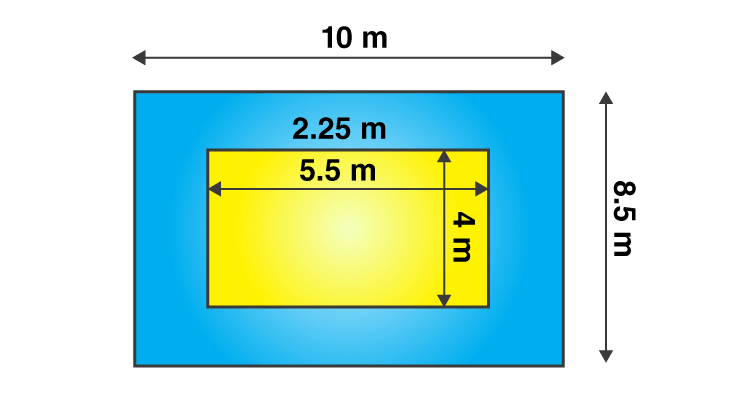# A verandah of width 2.25 m is constructed all along outside a room which is 5.5 m long and 4 m wide. Find: (i) the area of the verandah. (ii) the cost of cementing the floor of the verandah at the rate of ₹ 200 per m2.Given

From the given data we get to know that

Length of the cardboard (L) = 8 cm

Breadth of the cardboard (B) = 5 cm

Find out

(i) the area of the verandah. (ii) the cost of cementing the floor of the verandah at the rate of ₹ 200 per m2.

Solution

Area of the room = length × breadth

= 5.5 × 4

= 22 m2

From the figure,

The new length and breadth of the room when verandah is included is 10 m and 8.5 m respectively.

A new area of the room when verandah is included = 10 × 8.5

= 85 m2

The area of verandah = Area of the room when verandah is included – Area of the room

= 85 – 22

= 63 m2

(ii) The cost of cementing the floor of the verandah at the rate of ₹ 200 per m2

Then the cost of cementing the 63 m2 area of the floor of the verandah = 200 × 63

= ₹ 12600

Are of the margin=30 cm2(0)(0)